# Coordinate Geometry | NCERT Solutions Practice Questions | Maths

### Coordinate Geometry

Coordinate geometry is one of the most important and exciting ideas of mathematics. In particular it is central to the mathematics students meet at school. It provides a connection between algebra and geometry through graphs of lines and curves.

### Cartesian System

A Cartesian coordinate system is a coordinate system that specifies each point uniquely in a plane by a pair of numerical coordinates, which are the signed distances from the point to two fixed perpendicular directed lines, measured in the same unit of length.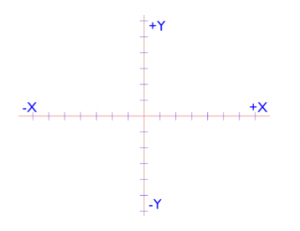Here +x indicates about positive x-axis and –x indicates negative x-axis.
Similarly +y indicates positive y-axis and –y indicates negative y-axis.

### Quadrants

You have observed that the axes have divided the plane into four parts. These four parts are called as quadrants which are numbered as I, II, III and IV anticlockwise from +x. So the plane consists of the axes and quadrants. We call the plane, the Cartesian plane or the coordinate plane or xy plane and the axis are called as coordinate axis.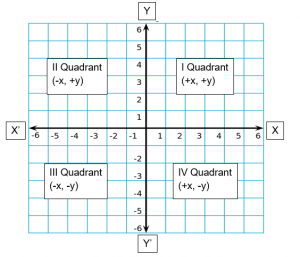Example 1

In which quadrant does the point P(-2, 3) lie?

Solution

Coordinate axes divide the plane of the graph paper into four quadrants.

In Quadrant I, sign of coordinates are (+, +)

In Quadrant II, sign of coordinates are (-, +)

In Quadrant III, sign of coordinates are (-, -)

In Quadrant IV, sign of coordinates are (+, -)

Since, In Point P (-2, 3) x-coordinate is negative while y-coordinate is positive.

Hence, Point P (-2, 3) lie in Quadrant II.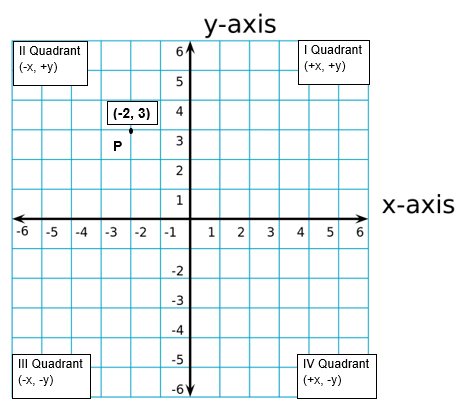Example 2

In which quadrant does the point Q (3, -4) lie?

Solution

Coordinate axes divide the plane of the graph paper into four quadrants.

In Quadrant I, sign of coordinates are (+, +)

In Quadrant II sign of coordinates are (-, +)

In Quadrant III sign of coordinates are (-, -)

In Quadrant IV sign of coordinates are (+, -)

Since, In Point Q (3, -4) x-coordinate is positive while y-coordinate is negative.

Hence, Point Q (3, -4) lie in Quadrant IV.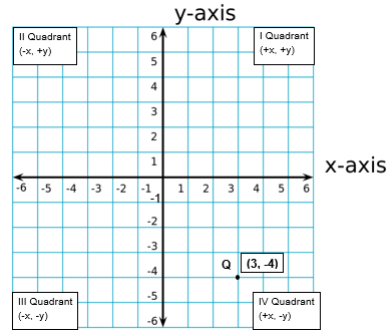Example 3

In which quadrant does the point O (-3, -2) lie?

Solution

Coordinate axes divide the plane of the graph paper into four quadrants.

In Quadrant I, sign of coordinates are (+, +)

In Quadrant II sign of coordinates are (-, +)

In Quadrant III sign of coordinates are (-, -)

In Quadrant IV sign of coordinates are (+, -)

Since, In Point O (-3, -2) both x and y coordinate are negative.

Hence, Point O (-3, -2) lie in Quadrant III.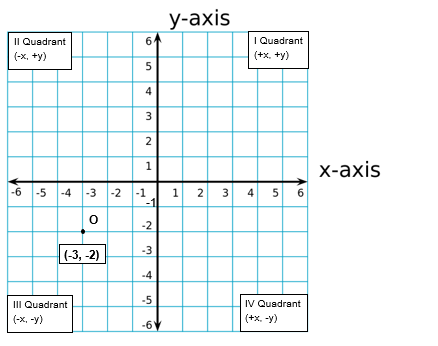Example 4

In which quadrant does the point P(1, 4) lie?

Solution

Coordinate axes divide the plane of the graph paper into four quadrants.

In Quadrant I, sign of coordinates are (+, +)

In Quadrant II, sign of coordinates are (-, +)

In Quadrant III, sign of coordinates are (-, -)

In Quadrant IV, sign of coordinates are (+, -)

Since, In Point P (1, 4) both x and y coordinate are positive

Hence, Point P (1, 4) lie in Quadrant I.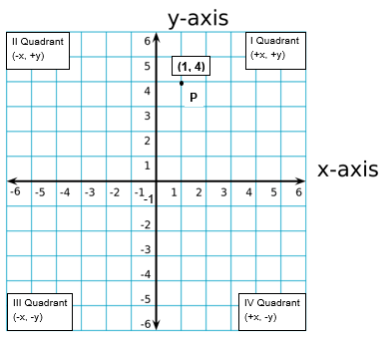#### How do we write the coordinates of a point?

We write the coordinates of a point using the following instruction.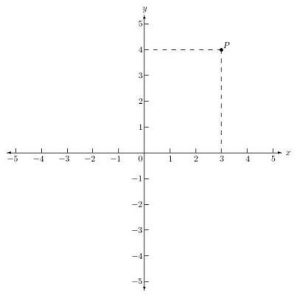1) The x-coordinates of a point is its perpendicular distance from y-axis measured along x-axis (Positive along the positive x-axis and Negative along the negative x-axis). For the point P it is 3. The X-coordinate is also called as the abscissa.

2) The y coordinates of a point is its perpendicular distance from x-axis measured along the y axis (Positive along the positive y-axis and negative along the negative y-axis). For the point P that will be 4. Y-coordinate is also known as the ordinate.

3) In stating the coordinates of a point in the coordinate plane, the x-coordinate comes first and then the y-coordinate. We place the coordinates in the bracket i.e, (3,4)

Example 1

Write the coordinates of point P.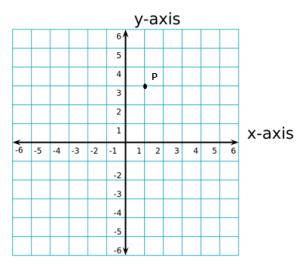Solution

The point P is at 1 unit distance from y-axis and 3 units distance from x-axis. Therefore point P is (1,3)

Example 2

Write the coordinates of point B.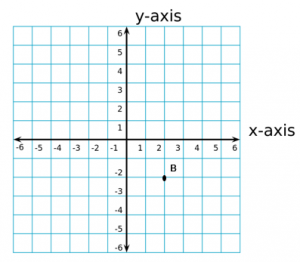Solution

Point is at 2 unit distance from y-axis along positive x-axis so the x coordinate is 2 and from x-axis along negative y-axis the distance is 2 so the y-coordinate is -2. So the point B will be (2, -2).

Example 3

Write the coordinates of point Q.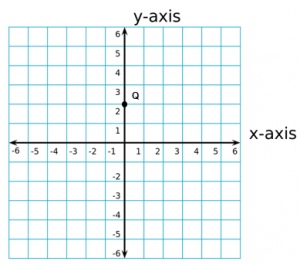Solution

The point is on y-axis so the distance from y-axis along the x-axis will be zero because it is on the y-axis. So the x coordinate will be zero. Similarly, the distance from x-axis along positive y-axis is 2 so the y-coordinate will be 2. So point Q is (0,2)

### How to Locate the points on the coordinate axis?

Let the coordinates of a point M be (-3, 2). We want to plot this point in the coordinate plane. We draw the coordinate axis and choose our units such that one centimeter represents one unit on both the axes. The coordinates of the point (-3, 2)tell us that the distance of this point from y axis along negative x-axis is 3 units and the distance of the point from the x-axis along the positive y-axis is 2 units. Starting from the origin we count 3 units on the negative x-axis and mark the corresponding point. Now starting from this point we move towards positive y axis and count 2 unit distance and mark the point. This point will be (-3, 2).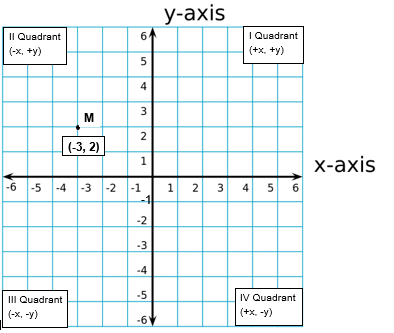### 1 thought on “Coordinate Geometry | NCERT Solutions Practice Questions | Maths”

1.I spent a lot of time to find something such as this

Reply